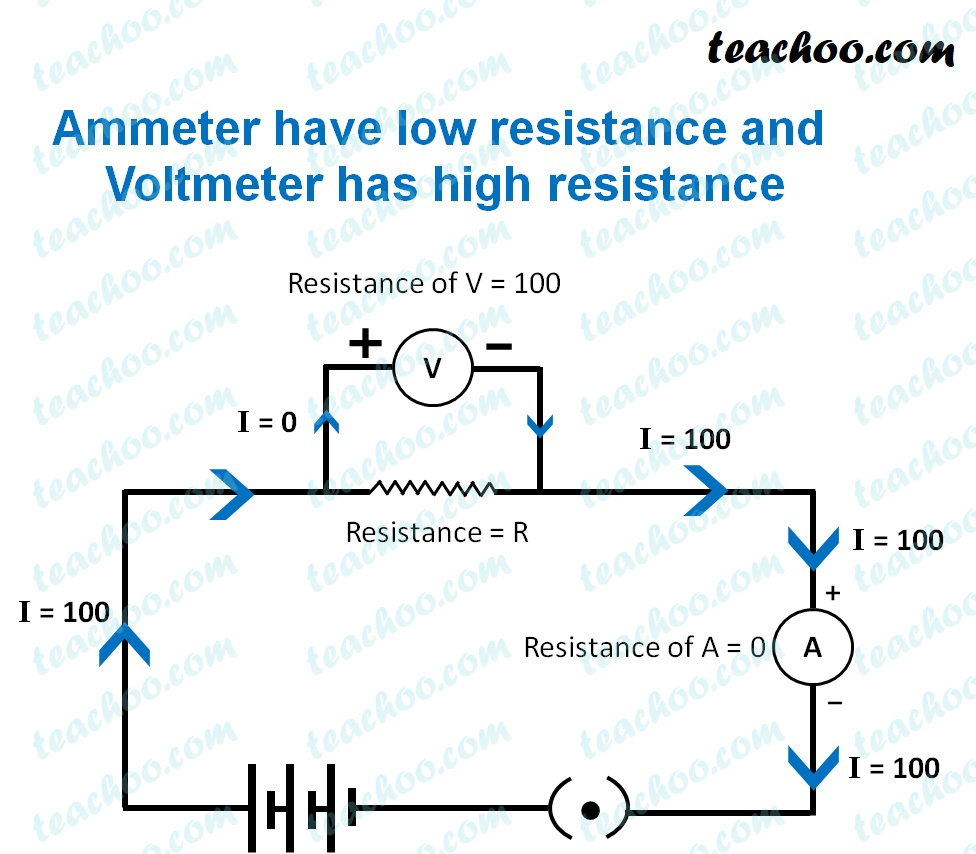Teachoo Questions

Class 10
Chapter 12 Class 10 - ElectricityAn ammeter is a device which measures the amount of current flowing in a circuit.

It is a very low resistance(nearly zero) device.

If it will be connected in parallel, it would draw most of the current and would get damaged. Hence, it is connected in series.

A voltmeter is a device which measures the amount of potential difference across a resistor or a series of resistors.

It is a very high resistance(nearly infinite) device.

If it will be connected in series, it would would increase the equivalent resistance of the circuit to nearly infinite and no current would

flow through the circuit.

Hence, it is connected in parallel.

Get live Maths 1-on-1 Classs - Class 6 to 12